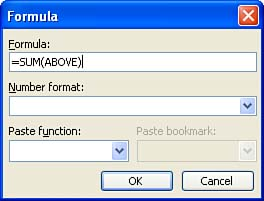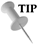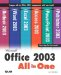# Placing a Formula in a Table

You can also place formulas in your tables that can do a variety of calculations. You can add cells to show a total, calculate an average, and do statistical calculations such as max and min, which return the maximum or minimum (respectively) from a list of numbers .

Before working with formulas, however, you should become familiar with how cell addresses are determined. Each column in the table is given a letter designation starting with A. So, the first column is A, the second is B and so on. The first row in the table is 1. The second row would be 2 and so on. The first cell in a table, which is in column A and row 1 would be cell A1. Each cell 's address is the column letter followed by the row number.

To specify a group of cells to be acted on by a formula, you have to specify the first cell in the group and then the last cell in the group . So if you were adding cells B2, B3, B4, and B5, you would designate the cell group as B2:B5. This tells Word where the cells to act on begin and end.

Another thing you should know is that table formulas have to be created in a very specific way. Each formula must start with an equal sign (=) followed by the name of the formula (such as SUM for adding or AVERAGE for average). The cells to be acted on are then entered in parentheses following the name of the formula. A formula that provides you the average of cells B2:B5 would look like this:

=AVERAGE(B2:B5)

To place a formula in a table, follow these steps:

1. Click in the table cell where you will place the formula.

2. Select Table , Formula . The Formula dialog box appears as shown in Figure 16.6.

##### Figure 16.6. The Formula dialog box allows you to insert a formula into a Word table.3. In many cases, the Formula dialog box will use cell position to interpret the best formula to insert into the table. For example, in Figure 16.6, Word "sees" that you have a column of numbers above the formula cell and so will probably want to add (SUM) cells above. If this is the appropriate formula, click OK and the formula (and its result) will be inserted into the table.

4. If you want to paste a different formula into the table cell, select and Delete the formula provided.

5. In the Formula box, type an equal sign ( = ) and then click the Paste Function drop-down list to insert a new formula.

6. To specify the cells to be acted on by the formula, place the starting and ending addresses of the cell grouping (separated by a colon ).

7. Click OK to place the formula in the table.Understanding Word Formulas The formulas that Word provides for use in your tables are simplified versions of Excel functions. For more about how functions work and are used, see Lesson 5, "Performing Calculations with Functions," in the Excel section of this book.Microsoft Office 2003 All-in-One
ISBN: B005HKSHB2
EAN: N/A
Year: 2002
Pages: 660
Authors: Joe Habraken

Similar book on Amazon